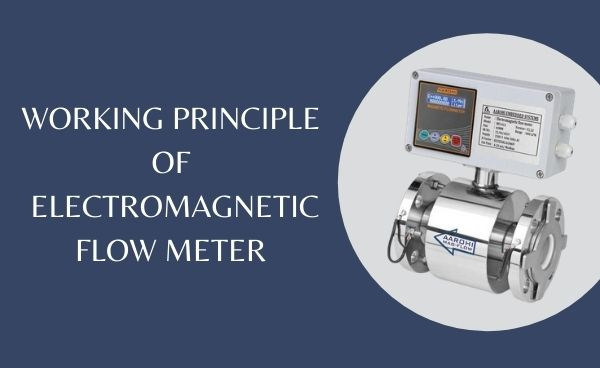# Electromagnetic Flow Meter Measuring Principle# What is an Electromagnetic Flow Meter

Electromagnetic Flow Meter, simply known as magflow meter is a volumetric flow meter. It is a transducer that measures fluid flow by the voltage induced across the liquid by its flow through a magnetic field.

A magnetic field is applied to the metering or flow tube, which results in a potential difference proportional to the flow velocity perpendicular to the flux lines.

The MAGFLOW (Standard Version) can be used for flow measurement in both the direction.

The MAGFLOW also gives us a DC Current Output in the range of 4-20mA proportional to flow before delivery the measuring range is set. Indication of the flow and adjust to suit customer requirements.

Recording instruments like current indicators, communication with power panel instrument,s and flow rate indicator can be connected with MAGFLOW output.

## Measuring Principal

Magnetic flow meters works based on Faraday’s Law of Electromagnetic Induction.

According to this principle, when a conductive medium passes through a magnetic field B, a voltage E is generated which is proportional to the velocity v of the medium, the density of the magnetic field, and the length of the conductor.

In a magnetic flow meter, a current is applied to wire coils mounted within or outside the meter body to generate a magnetic field.

The liquid flowing through the pipe acts as the conductor and this induces a voltage which is proportional to the average flow velocity.

This voltage is detected by sensing electrodes mounted in the Magflow meter body and sent to a transmitter which calculates the volumetric flow rate based on the pipe dimensions.

Mathematically,

we can state Faraday’s law as

E is proportional to V x B x L

where,

E – is the voltage generated in a conductor,

V- is the velocity of the conductor,

B- is the magnetic field strength.

L- is the length of the conductor.

### Basic working of magnetic flow meter

It is very important that the liquid flow that is to be measured using the magnetic flow meter must be electrically conductive. Faraday’s Law indicates that the signal voltage (E) is dependent on the average liquid velocity (V), the length of the conductor (D), and the magnetic field strength (B).

The Magnetic field will thus be established in the cross-section of the tube. Basically, when the conductive liquid flows through the magnetic field, voltage is induced. To measure this generated voltage (which is proportional to the velocity of the flowing liquid), two stainless steel electrodes are used which are mounted opposite each other.

The two electrodes which are placed inside the flow meter are then connected to an advanced electronic circuit that has the ability to process the signal.

The processed signal is fed into the microprocessor that calculates the volumetric flow of liquid.

to know more watch below video for 3D illustration of Electromagnetic flow meter working

## Magnetic Flow Meter Application

The main applications can be found in the following sectors,

• Water and wastewater
• Chemical and pharmaceutical industries
• Food & beverage industry
• Mining and cement industries
• Pulp and paper industry
• Steel industry
• Ceramic industries
• Power generation; utility and chilled water industry

## Advantages of magnetic flow meter

There are numerous benefits to using electromagnetic flowmeters to perform fluid flow measurements.

• No moving/rotary parts in line therefore there is no additional pressure losses is generated.
• Only the lining of the pipe wall and the electrodes are in contact with the measured liquid.
• The generated voltage is exact linear function of the average flow velocity.
• The signal from the entire space filled by the magnetic field, it occurs as an average value over the pipe section.
• They can usually measure multidirectional flow, either upstream or downstream.
• No moving/rotary parts in line therefore there is no additional pressure losses is generated.
• Only the lining of the pipe wall and the electrodes are in contact with the measured liquid.
• The generated voltage is exact linear function of the average flow velocity.
• The signal from the entire space filled by the magnetic field, it occurs as an average value over the pipe section.
• They can usually measure multidirectional flow, either upstream or downstream.

1.Informative and knowledgeable article about Electromagnetic flow meter.

1.Thank you very much, sir, for your valuable feedback keep learning.

2.Nicely explained!!!

1.Thank you very much, sir, for your valuable feedback keep learning.